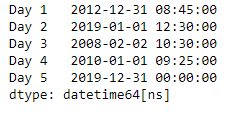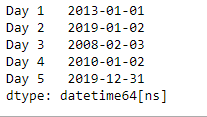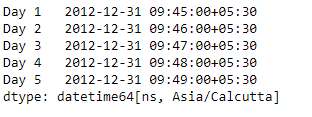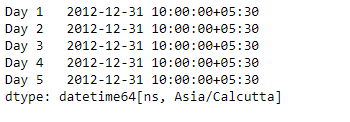# Python | Pandas Series.dt.ceil

`Series.dt` can be used to access the values of the series as datetimelike and return several properties. Pandas` Series.dt.ceil()` function perform ceil operation on the data to the specified freq.

Syntax: Series.dt.ceil(*args, **kwargs)

Parameter :
freq : The frequency level to ceil the index to

Returns : DatetimeIndex, TimedeltaIndex, or Series

Example #1: Use `Series.dt.ceil()` function to ceil the datetime data of the given series object to the specified frequency.

 `# importing pandas as pd ` `import` `pandas as pd ` ` `  `# Creating the Series ` `sr ``=` `pd.Series([``'2012-12-31 08:45'``, ``'2019-1-1 12:30'``, ``'2008-02-2 10:30'``, ` `               ``'2010-1-1 09:25'``, ``'2019-12-31 00:00'``]) ` ` `  `# Creating the index ` `idx ``=` `[``'Day 1'``, ``'Day 2'``, ``'Day 3'``, ``'Day 4'``, ``'Day 5'``] ` ` `  `# set the index ` `sr.index ``=` `idx ` ` `  `# Convert the underlying data to datetime  ` `sr ``=` `pd.to_datetime(sr) ` ` `  `# Print the series ` `print``(sr) `

Output :Now we will use `Series.dt.ceil()` function to ceil the datetime values in the given series object to Daily frequency.

 `# ceil to daily frequency ` `result ``=` `sr.dt.ceil(freq ``=` `'D'``) ` ` `  `# print the result ` `print``(result) `

Output :As we can see in the output, the `Series.dt.ceil()` function has successfully ceiled the datetime values in the given series object to the specified frequency.

Example #2 : Use `Series.dt.ceil()` function to ceil the datetime data of the given series object to the specified frequency.

 `# importing pandas as pd ` `import` `pandas as pd ` ` `  `# Creating the Series ` `sr ``=` `pd.Series(pd.date_range(``'2012-12-31 09:45'``, periods ``=` `5``, freq ``=` `'T'``, ` `                            ``tz ``=` `'Asia / Calcutta'``)) ` ` `  `# Creating the index ` `idx ``=` `[``'Day 1'``, ``'Day 2'``, ``'Day 3'``, ``'Day 4'``, ``'Day 5'``] ` ` `  `# set the index ` `sr.index ``=` `idx ` ` `  `# Print the series ` `print``(sr) `

Output :Now we will use `Series.dt.ceil()` function to ceil the datetime values in the given series object to Hourly frequency.

 `# ceil to hourly frequency ` `result ``=` `sr.dt.ceil(freq ``=` `'H'``) ` ` `  `# print the result ` `print``(result) `

Output :As we can see in the output, the `Series.dt.ceil()` function has successfully ceiled the datetime values in the given series object to the specified frequency.

My Personal Notes arrow_drop_upCheck out this Author's contributed articles.

If you like GeeksforGeeks and would like to contribute, you can also write an article using contribute.geeksforgeeks.org or mail your article to contribute@geeksforgeeks.org. See your article appearing on the GeeksforGeeks main page and help other Geeks.

Please Improve this article if you find anything incorrect by clicking on the "Improve Article" button below.

Article Tags :

Be the First to upvote.

Please write to us at contribute@geeksforgeeks.org to report any issue with the above content.